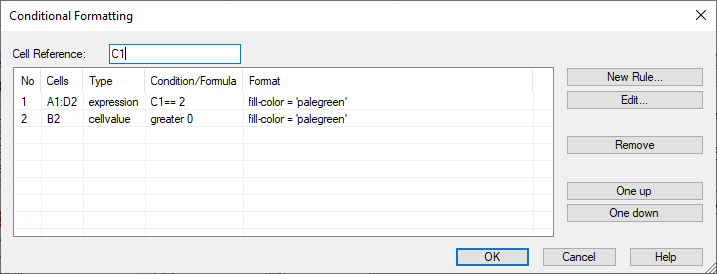# Edit=>Conditional Formatting¶

Conditional formatting can be used to change the text color and fill color of a table cell depending on the cell’s value, or to create a rule with a formula.

To add a new conditional formatting rule to a table:

Execute the command:

• Select the cell range to apply the cell formatting,
• Right click on the selected cell range and choose Conditional Formatting=>Add Cell RuleCell Reference

Specifies the cells reference in the A1-Style (column letter and row number). It refers to a single cell, for example B2 or a ranges of cells, for example A1:C4. The cell reference specifies the cells to which the format is applied.

Format all cells based on their values

If enabled, the cell value will be compared to the specified value Value1. The following is of operators that can be used:

Operator
greater as (>)
greater or equal (>=)
less as (<)
less or equal (<=)
between (>= v1 && < v2)
not between (< v1 || > v2)
equal (==)
not equal (!=)
Value1
Is a real value.
Value2
Is a real value. Will only be used with `between` and `not between`.

Format all cells based on the formula expression

Expression

In an expression the following operators can be used: `>`, `>=`, `<`, `>=`, `==`, `!=`. The expressions can be AND (`&&`) and OR (`||`) connected.

Liste mit Beispielen:

Expression Description
`A1 > 10` If the value in cell A1 is greater as 10, the expression is TRUE (1).
`B2 > C2` If the value in B2 is greater than the value in C2, the expression is TRUE (1).
`sum(B2:E2) <= 10` If the sum of the cells in the range B2 to E2 is smaller or equal to 10, the expression is TRUE (1).
`A1 > B1 && A1 < C2` If the value in cell A1 is between the values of B1 and C2, the expression is TRUE (1).

Fill Color

Color ist used to fill the cell.

** Font Color**

Color is used to display the cell value.

# Condional Format Dialogbox¶

The following dialog box can be used to add, remove or edit cell rules.List of cell rules.

Each row is a format rule. The rules are evaluated as shown in the list.

# UniScript-Interface¶

The TableFormatCondition_AddFormat function can be used in UniScript to add conditional formatting to a table. The format parameter is an object Example:

```oFormat = [. sqref = "a1:a2",
type = "cellvalue",
formula = ["less", "0"],
format = "fill-color = 'cyan' font-color = 'red'"];
```

The color can also be specified as an RGB value, as follows:

```oFormat.format = "fill-color = 'rgb(255,0,0)' font-color = 'rgb(100,100,100)'";
```

Operator names: greater, between, notbetween, greaterequal, less, lessequal, equal, notequal.

More examples:

```oFormat = [. sqref = "a1", type = "cellvalue",  formula = ["between", "10", "20"], format = fill-color='rgb(255,0,0)'];
oFormat = [. sqref = "a1", type = "cellvalue",  formula = ["between", "10", "20"], format = fill-color='rgb(255,0,0)'];
oFormat = [. sqref = "C4:G4",  type = "expression", formula = "(C4 < D4)",  format = fill-color='green'];
oFormat = [. sqref = "D9:G13", type = "expression", formula = "(D9/1000 > 0.46)", format = fill-color='rgb(255,0,0)'];
```

History

Version Description
R2018.6 New.

id-1237658